RRB NTPC Mock Test - 2 (English)

# RRB NTPC Mock Test - 2 (English)

Test Description

## 100 Questions MCQ Test RRB NTPC Mock Tests Series | RRB NTPC Mock Test - 2 (English)

RRB NTPC Mock Test - 2 (English) for Railways 2023 is part of RRB NTPC Mock Tests Series preparation. The RRB NTPC Mock Test - 2 (English) questions and answers have been prepared according to the Railways exam syllabus.The RRB NTPC Mock Test - 2 (English) MCQs are made for Railways 2023 Exam. Find important definitions, questions, notes, meanings, examples, exercises, MCQs and online tests for RRB NTPC Mock Test - 2 (English) below.
Solutions of RRB NTPC Mock Test - 2 (English) questions in English are available as part of our RRB NTPC Mock Tests Series for Railways & RRB NTPC Mock Test - 2 (English) solutions in Hindi for RRB NTPC Mock Tests Series course. Download more important topics, notes, lectures and mock test series for Railways Exam by signing up for free. Attempt RRB NTPC Mock Test - 2 (English) | 100 questions in 90 minutes | Mock test for Railways preparation | Free important questions MCQ to study RRB NTPC Mock Tests Series for Railways Exam | Download free PDF with solutions
 1 Crore+ students have signed up on EduRev. Have you?
RRB NTPC Mock Test - 2 (English) - Question 1

### What is the full form of LTE with respect to mobile communication?

Detailed Solution for RRB NTPC Mock Test - 2 (English) - Question 1
• LTE is a standard for wireless communication of high-speed data for mobile phones and data terminals.
• It is based on the GSM/EDGE and UMTS/HSPA network technologies
RRB NTPC Mock Test - 2 (English) - Question 2

### In question, select the pair which is different from other three alternatives.

Detailed Solution for RRB NTPC Mock Test - 2 (English) - Question 2

All except Allow-permit are antonyms pairs. Allow is synonyms of permit.

Hence, Allow-Permit is the odd pair

RRB NTPC Mock Test - 2 (English) - Question 3

### A series is given with one term missing. Select the correct alternative from the given ones that will complete the series. B, E, I, N, ?

Detailed Solution for RRB NTPC Mock Test - 2 (English) - Question 3

Given Series: B, E, I, N, ?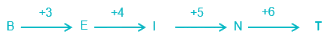The logic followed for the letters:

Hence, the next term will be T.

RRB NTPC Mock Test - 2 (English) - Question 4

Select the related letters from the given alternatives.
MSTU : NTVW ∷ LSPR : ?

Detailed Solution for RRB NTPC Mock Test - 2 (English) - Question 4

Relation in MSTU : NTVW is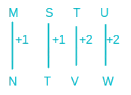Similarly;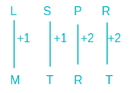Hence, “MTRT” is the correct answer

RRB NTPC Mock Test - 2 (English) - Question 5

20 kg of a mixture of wheat and husk contains 5% husk. How many kg more of husk must be added to make the husk content 20% in the new mixture?

Detailed Solution for RRB NTPC Mock Test - 2 (English) - Question 5

Given, Husk in 20 kg of mixture = 5% of 20 = 1 kg

Let x kg husk is been added

According to the question,

⇒ 1 + x = 20% of (20 + x)

⇒ 1 + x = (1/5) × (20 + x)

⇒ 5 + 5x = 20 + x

⇒ 4x = 15

⇒ x = 15/4 = 3.75

∴ 3.75 kg of husk must be added.

RRB NTPC Mock Test - 2 (English) - Question 6

Which of the following is a Biological method of soil conservation?

Detailed Solution for RRB NTPC Mock Test - 2 (English) - Question 6
• Contour farming is a biological method of soil conservation.
• This method is practiced in the hilly regions or on the slopes.
• Farming on the contour creates small ridges that slow runoff water, and it increases the rate of water infiltration, reduced the hazard of erosion.

Rest all are mechanical methods.

RRB NTPC Mock Test - 2 (English) - Question 7

Who was selected as the new President of the World Bank in April 2019?

Detailed Solution for RRB NTPC Mock Test - 2 (English) - Question 7
• American David Malpass is selected as the new President of the World Bank.
• David Malpass is currently the US Treasury Department's Under Secretary for International Affairs.
• He was a senior economic adviser to US President Donald Trump during his 2016 election campaign.
• Malpass succeeded Jim Yong-Kim, who stepped down on February 1 to join an investment firm.
RRB NTPC Mock Test - 2 (English) - Question 8

How many times the hands of the clock coincides in 12 hours.

Detailed Solution for RRB NTPC Mock Test - 2 (English) - Question 8

Here, the hands of the clock coincides for every 1 hour. But between 11 and 12 and 12 and 1, the hands of clock coincides only once that is at 12 : 00.

to prove this we have formula;

30(11) = (11/2)M । 30(0) = (11/2)M (Here zero is 12)

M = 660 / 11 । 0 = 11M

M = 60 । M = 0

i.e; 11 : 60 = 12 : 00 । i.e; 12 : 00

Hence, 11 times the hands of the clock coincides in 12 hours.

RRB NTPC Mock Test - 2 (English) - Question 9

Which of the following waves do not require any kind of medium to propagate?

Detailed Solution for RRB NTPC Mock Test - 2 (English) - Question 9

Electromagnetic waves are waves which can travel through the vacuum of outer space. Mechanical waves, unlike electromagnetic waves, require the presence of a material medium in order to transport their energy from one location to another.

Sound waves, Earthquake waves and Water surface waves are examples of mechanical waves while light waves are examples of electromagnetic waves.

RRB NTPC Mock Test - 2 (English) - Question 10

Who is the author of the book, ‘The Making of a Legend’?

Detailed Solution for RRB NTPC Mock Test - 2 (English) - Question 10

‘The Making of a Legend’ is written by Sulabh International Chief Bindeshwar Pathak. The book chronicles the life of Prime Minister Narendra Modi. The book was released by RSS chief Mohan Bhagwat on July 12.

RRB NTPC Mock Test - 2 (English) - Question 11

A copper wire when bent in the form of a square encloses an area of 121 cm2. If the same wire is bent into the form of a circle, find the area of the circle. (Use π = 22/7)

Detailed Solution for RRB NTPC Mock Test - 2 (English) - Question 11

Area of square = 121 cm2

Let the side of square be ‘a’ cm,

⇒ a= 121 cm2

⇒ a = 11 cm

Now, the length of the wire = Perimeter of square

⇒ Length = 4 × 11 = 44 cm

Also, Length of wire = Perimeter of circle

⇒ 2πr = 44 cm

⇒ 2 × 22/7 × r = 44 cm

⇒ r = 7 cm

Area of circle = πr2

∴ Area = 22/7 × 7 × 7 = 154 cm2

RRB NTPC Mock Test - 2 (English) - Question 12

Which of the following is the plant hormone that induces cell division?

Detailed Solution for RRB NTPC Mock Test - 2 (English) - Question 12

Cytokinin is the plant hormone that induces cell division. It also plays an important role in coordination of many growth and behavioural processes in the plant's life cycle.

RRB NTPC Mock Test - 2 (English) - Question 13

Which of the following gases has the highest solubility in water at room temperature?

Detailed Solution for RRB NTPC Mock Test - 2 (English) - Question 13

100 gm of water can dissolve as much as 52.9 gm of ammonia at 20 degree Celsius and 1 atm pressure. A solution of ammonia in water is called ammonium hydroxide, NH4OH. Ammonia's high solubility in water is due to hydrogen bonding with water molecules.

RRB NTPC Mock Test - 2 (English) - Question 14

The addition of a rational number and its reciprocal is 61/30. Calculate the number.

Detailed Solution for RRB NTPC Mock Test - 2 (English) - Question 14

Let the rational number be x

x + (1/x) = 61/30

⇒ 30x2 - 61x + 30 = 0

⇒ 30x2 - 25x - 36x + 30 = 0

⇒ 5x(6x - 5) - 6(6x - 5) = 0

⇒ (6x - 5)(5x - 6) = 0

∴ x = 5/6, 6/5

RRB NTPC Mock Test - 2 (English) - Question 15

Which of the following laws does not relate to gases?

Detailed Solution for RRB NTPC Mock Test - 2 (English) - Question 15
• Len'z law is not related to gases.
• Lenz's law is a common way to understand how electromagnetic circuits obey Newton's third law and the conservation of energy. It is named after Heinrich Lenz and says: an induced electromotive force always gives rise to a current whose magnetic field opposes the change in original magnetic flux.
RRB NTPC Mock Test - 2 (English) - Question 16

The famous poem ‘Padmavat’ describing story of Alauddin Khilji and Rani Padmini was written by ________.

Detailed Solution for RRB NTPC Mock Test - 2 (English) - Question 16

Padmavat is an epic poem written in 1540 by Sufi poet Malik Muhammad Jayasi, who wrote it in the Hindustani language of Awadhi, and originally in the Persian Nastaʿlīq script. It is the oldest extant text among the important works in Awadhi.

RRB NTPC Mock Test - 2 (English) - Question 17

Goldilocks zone is associated with which of the following?

Detailed Solution for RRB NTPC Mock Test - 2 (English) - Question 17

Goldilocks region is an area of space in which a planet is just the right distance from its home star so that its surface is neither too hot nor too cold. Earth lies in the Goldilocks zone.

RRB NTPC Mock Test - 2 (English) - Question 18

Direction: Arrange the given words in the order in which they will be arranged in a dictionary and choose the one that comes fourth.
Native, Narrow, Nation, Natural

Detailed Solution for RRB NTPC Mock Test - 2 (English) - Question 18

In all the words “Na” is in common. The next letters are r, t.

So Narrow comes first.

And “Nat” is common in Native, Nation and Natural.

So in this the letter “i” comes first than “u”, but with “ï” again we have two words those are Native and Nation. So here “Nati” is common.

Consider the next letter that is “o “ comes first than “v“. Hence Nation will be the second word and Native will be third.

And Natural will be fourth.

Finally, dictionary order:

Narrow, Nation, Native, Natural.

Hence, Natural becomes fourth.

RRB NTPC Mock Test - 2 (English) - Question 19

Find the wrong term in the series.
C, E, G, Q, U

Detailed Solution for RRB NTPC Mock Test - 2 (English) - Question 19

The series consists of prime position of alphabet.

C = 3

E = 5

G = 7

Q = 17

U = 21 (this is not a prime position)

So, the wrong term is U.

RRB NTPC Mock Test - 2 (English) - Question 20

Ramesh walks 8 km towards the north. From there he takes a right turn and walks 9 km. Then he takes a left turn and walks 5 km. Finally, he takes a left turn and walks 12 km. In which direction is he now with respect to the starting point?

Detailed Solution for RRB NTPC Mock Test - 2 (English) - Question 20

As per the given information,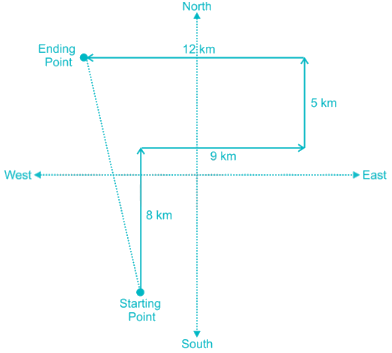Hence, Ramesh is now facing to the North-west direction, with respect to the starting point.

RRB NTPC Mock Test - 2 (English) - Question 21

In which of the following states India’s longest expressway has been inaugurated?

Detailed Solution for RRB NTPC Mock Test - 2 (English) - Question 21

The Agra Lucknow Expressway is a 302 km controlled-access highway or expressway, constructed by the Uttar Pradesh Expressways Industrial Development Authority. It is India’s longest expressway.

RRB NTPC Mock Test - 2 (English) - Question 22

In the following question, find out the alternative which will replace the question mark.
Fish : School ∷ ? : Army

Detailed Solution for RRB NTPC Mock Test - 2 (English) - Question 22

A group of fishes is known as School.

Similarly, a group of frogs is known as Army.

RRB NTPC Mock Test - 2 (English) - Question 23

Choose the word which is different from the rest.

Detailed Solution for RRB NTPC Mock Test - 2 (English) - Question 23

Alluvial, Regur and Laterie are types of soil

Whereas clay is the component of soil.

Hence, Clay is the odd term.

RRB NTPC Mock Test - 2 (English) - Question 24

Direction: The given pie chart represents the annual yield of certain crops in tons (in degree). Study the chart carefully and answer the following question.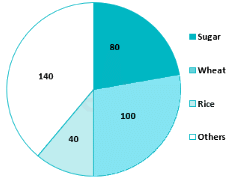Q. If the total production is 9000 tons, then the yield of rice in tons is.

Detailed Solution for RRB NTPC Mock Test - 2 (English) - Question 24

360° = 9000 tons

⇒ 1° = 25 tons

Now rice = 40°

⇒ rice = 40 × 25 = 1000

∴ yield of rice = 1000 tons

RRB NTPC Mock Test - 2 (English) - Question 25

Which of the following numbers represent triangle and circle only?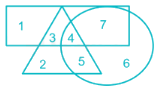Detailed Solution for RRB NTPC Mock Test - 2 (English) - Question 25

1) 3 → represents triangle and rectangle.

2) 7 → represents circle and rectangle.

3) 4 and 5 → 4 represents triangle, circle and rectangle, whereas 5 represents triangle and circle.

As number which represents triangle and circle is number 5.

RRB NTPC Mock Test - 2 (English) - Question 26

Which of the following books had Chand Bardai written?

Detailed Solution for RRB NTPC Mock Test - 2 (English) - Question 26

Chand Bardai was an Indian poet, who composed Prithviraj Raso, an epic poem in Brajbhasa about the life of the Chahamana king Prithviraj Chauhan. The poem presents him as a court poet of Prithviraj.

RRB NTPC Mock Test - 2 (English) - Question 27

Which of the following is the chemical name of common salt?

Detailed Solution for RRB NTPC Mock Test - 2 (English) - Question 27

Sodium Chloride is the chemical name of common salt. It is formed when Sodium and Chlorine react with each other to give this salt and water.

RRB NTPC Mock Test - 2 (English) - Question 28

The shadow of a tower standing on a level ground is found to be 40 metre longer when the suns altitude is 30° than when it is 60°. Find the length of the tower.

Detailed Solution for RRB NTPC Mock Test - 2 (English) - Question 28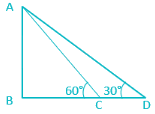We have CD = 40 m.

In ΔABC,

tan 60 = AB/BC

⇒ √3 = AB/BC

⇒ AB = √3 BC

Now, we have

In ΔABD,

⇒ tan 30 = AB/(BC + 40)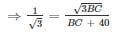⇒ BC + 40 = 3 BC

⇒ BC = 20 m.

Hence, AB = √3 BC

⇒ AB = 20√3 m.

RRB NTPC Mock Test - 2 (English) - Question 29

Roshan’s current age is 2 years less than 1.6 times that of Usha’s. 8 years ago, Usha’s age was 1 year more than half of Roshan’s age 8 years ago. What is Roshan’s present age in years?

Detailed Solution for RRB NTPC Mock Test - 2 (English) - Question 29

Let Roshan’s age be x and Usha’s age be y

Roshan’s current age is 2 years less than 1.6 times that of Usha’s,

⇒ x = 1.6y – 2

⇒ 8y – 5x = 10      ----(1)

8 years ago, Usha’s age was 1 year more than half of Roshan’s age 8 years ago,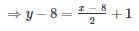⇒ 2y – x = 10

⇒ 8y – 4x = 40      ----(2)

From (1) and (2) we get,

⇒ 5x – 4x = 40 – 10

⇒ x = 30

∴ Roshan’s present age = 30 years

RRB NTPC Mock Test - 2 (English) - Question 30

Directions: In the question below is given a statement followed by two assumptions numbered I and II. You have to consider the statement and the following assumptions and decide which of the assumptions is implicit in the statement.

Statement: Neither my sister nor my brother is particularly interesting.

Assumption I: My brother is interesting.
Assumption II: My sister is more interesting than my brother.

Detailed Solution for RRB NTPC Mock Test - 2 (English) - Question 30

From the statement, it is clear that both the brother as well as sister are not interesting(boring). Hence both the assumptions are invalid as one says that the brother is interesting and the other talks about who is more interesting.

RRB NTPC Mock Test - 2 (English) - Question 31

Which answer figure will complete the pattern in the following question figure.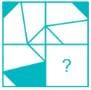Detailed Solution for RRB NTPC Mock Test - 2 (English) - Question 31The, downward part is reversed of the upper part. The portion shaded in upper part is not shaded in lower part and the part not shaded in upper part is shaded in lower part.

RRB NTPC Mock Test - 2 (English) - Question 32

What is the value of {(1/2) - cot45°}?

Detailed Solution for RRB NTPC Mock Test - 2 (English) - Question 32

∴ (1/2) - cot45° = (1/2) - 1 = -1/2

RRB NTPC Mock Test - 2 (English) - Question 33

The population of a town is 350000. It increases annually at the rate of 20%. What will be the population after 2 years?

Detailed Solution for RRB NTPC Mock Test - 2 (English) - Question 33

If the current population of a town is P and it increases at a rate of R% per annum, then,

Population after n years = P × (1 + R/100)2

Given, P = 350000, R = 20%,

∴ Population after 2 years = 350000 × (1 + 20/100)2 = 350000 × 1.44 = 504000

RRB NTPC Mock Test - 2 (English) - Question 34

A natural number, when divided by 3, 4, 6 and 7, leaves a remainder of 2 in each case. What is the smallest of all such numbers?

Detailed Solution for RRB NTPC Mock Test - 2 (English) - Question 34

⇒ LCM of 3, 4, 6 and 7 = 3 × 22 × 7 = 84

∴ The required number = 84 + 2 = 86

RRB NTPC Mock Test - 2 (English) - Question 35

Pointing to a girl in the food court, Prem said, “She is the daughter of the only son of my mother’s father”. How is the girl’s mother related to Prem’s mother?

Detailed Solution for RRB NTPC Mock Test - 2 (English) - Question 35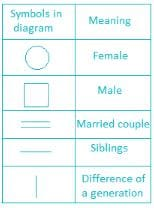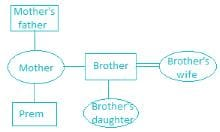Below table represents symbols used to draw a family tree,

According to the given information, we get the following tree diagram.

Hence, girl’s mother is sister-in-law to Prem’s mother.

RRB NTPC Mock Test - 2 (English) - Question 36

Which of the forces combine together to keep planets in their respective orbits?

Detailed Solution for RRB NTPC Mock Test - 2 (English) - Question 36

Gravitation and Centrifugal force combine together to keep planets in their respective orbits so that they don’t collide with each other.

RRB NTPC Mock Test - 2 (English) - Question 37

Which planet is the hottest planet in our solar system?

Detailed Solution for RRB NTPC Mock Test - 2 (English) - Question 37

Venus is the hottest planet in our solar system. The reason that Venus is hotter than Mercury is that it has an atmosphere made of carbon dioxide; it also has clouds of acid inside its atmosphere.

RRB NTPC Mock Test - 2 (English) - Question 38

In the following question, four number pairs are given. The number on left side of (-) is related to the number on the right side of (-) with some Logic/Rule/Relation. Three are similar on basis of same Logic/Rule/Relation. Select the odd one out from the given alternatives.

Detailed Solution for RRB NTPC Mock Test - 2 (English) - Question 38

1) 14 - 196 → 14 - 142

2) 16 - 256 → 16 - 162

3) 18 - 324 → 18 - 182

4) 22 - 464 → 22 - 464

Hence, 22 - 464 is the odd one out.

RRB NTPC Mock Test - 2 (English) - Question 39

Which is the longest bridge in India?

Detailed Solution for RRB NTPC Mock Test - 2 (English) - Question 39

The Bhupen Hazarika Setu or Dhola – Sadiya Bridge is a beam bridge in India, connecting the northeast states of Assam and Arunachal Pradesh. Its length is 9.15 kilometres (5.69 mi) in length and is the longest bridge in India.

• Bhupen Hazarika Setu length - 9.15km
• Bandra - Worli Sea Link length - 5.6 km.
• Mahatma Gandhi Setu length - 5.75km
• Vikramshila Setu length - 4.7 km
RRB NTPC Mock Test - 2 (English) - Question 40

The ratio of sand to gravel in a mixture is 17 : 8 while that between gravel and cement is 6 : 17. What is the ratio of sand to cement in the mixture?

Detailed Solution for RRB NTPC Mock Test - 2 (English) - Question 40

Ratio between sand and gravel = 17 : 8

Ratio between gravel and cement = 6 : 17

To get combined ratio, we can write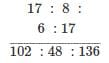∴ Sand ∶ Cement = 102 : 136 = 3 : 4

RRB NTPC Mock Test - 2 (English) - Question 41

What is the average of all even natural numbers upto 100?

Detailed Solution for RRB NTPC Mock Test - 2 (English) - Question 41

Average of numbers = Sum of numbers/ Total numbers

The numbers are 2, 4, 6, 8, 10…,100

Using the formula of AP, number of terms in an AP with difference ‘d’ and first term ‘a’ is given by

Nth term = a + (n - 1)d

Here N is 100, 100 = 2 + (n - 1)2 ⇒ n = 50

Sum of n terms of an AP = n/2(2a + (n - 1)d)

Here n is 50, sum = (50/2) × (2 × 2 + (49) × 2) = 2550

Sum of first 50 natural numbers = 2550

Average = 2550/50 = 51

RRB NTPC Mock Test - 2 (English) - Question 42

If the selling price of 10 articles is equal to the cost price of 15 articles, then what is the profit percent?

Detailed Solution for RRB NTPC Mock Test - 2 (English) - Question 42

Selling price = S.P

Cost price = C.P

Selling price of 10 article = cost price of 15 article

⇒ 10 S.P = 15 C.P

⇒ S.P = 1.5 C.P

Profit percentage = (profit/C.P) × 100 = {(S.P - C.P)/C.P} × 100 = {(1.5 C.P - C.P)/C.P} × 100 = 0.5 × 100 = 50%

RRB NTPC Mock Test - 2 (English) - Question 43

There are six students, P, Q, R, S, T and U who have a different number of pens - 2, 4, 5, 7, 9 and 12 (not necessarily in the same order). The number of pens Q has is a prime number. U has 2 more pens than Q. The number of pens R has is a multiple of 4 but not a multiple of 3. P has fewer pens than Q but has more pens than S. Who has the highest number of pens?

Detailed Solution for RRB NTPC Mock Test - 2 (English) - Question 43

Students: P, Q, R, S, T and U.

No. of pens: 2, 4, 5, 7, 9 and 12 (not necessarily in the same order).

1) The number of pens Q has is a prime number.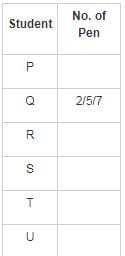2) U has 2 more pens than Q.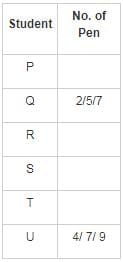3) The number of pens R has is a multiple of 4 but not a multiple of 3.

(So, R has 4 pens only.)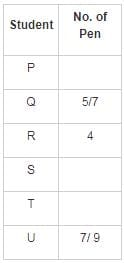4) P has fewer pens than Q but has more pens than S.

(P cannot have 2 pens as S has fewer pens than P.

So, P has 5 pens and S has 2 pens.)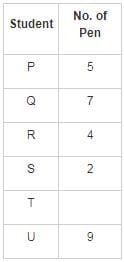(So, T has 12 pens.)

Final arrangement: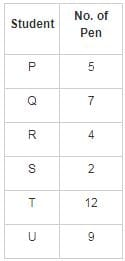Hence, T has the highest number of pens.

RRB NTPC Mock Test - 2 (English) - Question 44

Rearrange the jumbled-up letters in their natural sequence and find the odd one out.

Detailed Solution for RRB NTPC Mock Test - 2 (English) - Question 44

1) ROWARPS → SPARROW

2) TRSOICH → OSTRICH

3) ELAGE → EAGLE

4) EGONIP → PIGEON

Sparrow, Eagle and Pigeon are birds which can fly. But, Ostrich is a flightless bird.

Hence, “Ostrich” is the correct alternatives.

RRB NTPC Mock Test - 2 (English) - Question 45

Which of the following is the Controlling Centre of the Cell?

Detailed Solution for RRB NTPC Mock Test - 2 (English) - Question 45

The nucleus maintains the integrity of genes and controls the activities of the cell by regulating gene expression-the nucleus is, therefore, the controlling centre of the cell.

RRB NTPC Mock Test - 2 (English) - Question 46

ΔPQR ≅ ΔXYZ and PQ = 5.6 cm and XY = 8.4 cm. If perimeter of ΔXYZ = 24 cm, what will be the perimeter of ΔPQR?

Detailed Solution for RRB NTPC Mock Test - 2 (English) - Question 46

According to problem,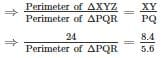⇒ Perimeter of ΔPQR = 24 × 5.6/8.4

⇒ Perimeter of ΔPQR = 16

RRB NTPC Mock Test - 2 (English) - Question 47

If Newspaper is Magazine, Magazine is Book, Book is Notebook, Notebook is Pencil, and Pencil is Eraser. What will be used for writing?

Detailed Solution for RRB NTPC Mock Test - 2 (English) - Question 47

For writing, we use pencil,

And here pencil is denoted by eraser.

Hence, ‘Eraser’ is the correct answer.

RRB NTPC Mock Test - 2 (English) - Question 48

Which of the following rocks is made up of lava and magma?

Detailed Solution for RRB NTPC Mock Test - 2 (English) - Question 48

Igneous rock is one of the three main rock types, the others being sedimentary and metamorphic. It is formed through the cooling and solidification of magma or lava.

RRB NTPC Mock Test - 2 (English) - Question 49

The Year of the Runaways was written by _________.

Detailed Solution for RRB NTPC Mock Test - 2 (English) - Question 49

A story about the lives of three Indian men and a British-Indian woman in the city puts the most urgent questions of the day in a human context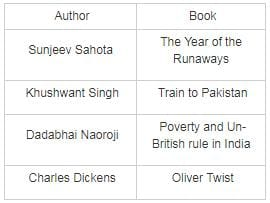RRB NTPC Mock Test - 2 (English) - Question 50

A man invested Rs. 2000 in a scheme offering 20% return at S.I for first 2 years and 25% return for next 2 years and 15% return for next 3 years. Find total interest received.

Detailed Solution for RRB NTPC Mock Test - 2 (English) - Question 50

Interest received for first 2 years = (2000 × 20 × 2)/100 = 800

Interest received for next 2 years = (2000 × 25 × 2)/100 = 1000

Interest received for next 3 years = (2000 × 15 × 3)/100 = 900

Total interest received = 800 + 1000 + 900 = 2700

RRB NTPC Mock Test - 2 (English) - Question 51

Ghoomar is a folk dance of ________.

Detailed Solution for RRB NTPC Mock Test - 2 (English) - Question 51

Ghoomar is a traditional folk dance of Rajasthan, India and Sindh‚ Pakistan. The dance is chiefly performed by veiled women who wear flowing dresses called ghaghara. The dance typically involves performers pirouetting while moving in and out of a wide circle. The word ghoomna describes the twirling movement of the dancers and is the basis of the word ghoomar. Ghoomar is often performed on special occasions, such as at weddings and during Holi and sometimes lasts for hours.

RRB NTPC Mock Test - 2 (English) - Question 52

Which of the following rivers originate from Aravalli ranges?

Detailed Solution for RRB NTPC Mock Test - 2 (English) - Question 52

River Sabarmati originates from the Aravalli ranges in the Udaipur district of Rajasthan and ends in the Gulf of Khambat of Arabian Sea.

RRB NTPC Mock Test - 2 (English) - Question 53

Directions: In making decisions about important questions, it is desirable to be able to distinguish between strong and weak arguments. Strong arguments are those, which are both important and directly related to the question. Weak arguments are those, which are of minor importance and also may not be directly related to the question or may be related to a trivial aspect of the question. The question below is followed by two arguments numbered I and II. You have to decide which of the arguments, strong argument is and which weak argument is.

Statement: Should the Indian government free all the prisoners of war?

Arguments:
I. No, they are the proof of bravery, power, and strength of the army.
II. Yes, war is over they are civilians with freedom and rights.

Detailed Solution for RRB NTPC Mock Test - 2 (English) - Question 53

Once a war is over the prisoners of war cannot be used as 'the proof of bravery, power, and strength of the army'. Argument I is vague and hence does not follow.

Argument 1 states that once the war is over the prisoners of war should be freed as they are 'are civilians with freedom and rights' which is a strong reason, hence argument II follows.

RRB NTPC Mock Test - 2 (English) - Question 54

In the following questions, in four out of the given five pairs of figures, figure I is related to figure II in the same particular manner. Spell out the pair in which this relationship does not exist between figure I and II.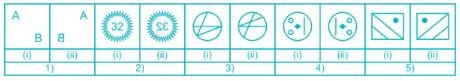Detailed Solution for RRB NTPC Mock Test - 2 (English) - Question 54

In each pair of I and II figure the II figure is a mirror image of figure I.

In the figure given below, the figure II is not the mirror image of figure I.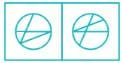Hence, option 4) represents the correct answer.

RRB NTPC Mock Test - 2 (English) - Question 55

In the following question, select the number which can be placed at the sign of question mark (?) from the given alternatives.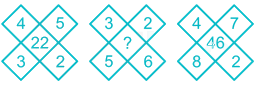Detailed Solution for RRB NTPC Mock Test - 2 (English) - Question 55

The pattern followed here is:

4 × 3 = 12; 5 × 2 = 10, 12 + 10 = 22

3 × 5 = 15; 2 × 6 = 12, 15 + 12 = 27

So, 2 × 7 = 14; 8 × 4 = 32, 14 + 32 = 46

Thus, 27 is the correct answer.

RRB NTPC Mock Test - 2 (English) - Question 56

In the following figure Rectangle represents Bake, Circle represents Grill, Triangle represents roast, and Square represents fry. Which set of letter(s) represent(s) grill and roast but not fry.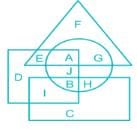Detailed Solution for RRB NTPC Mock Test - 2 (English) - Question 56

1) Rectangle represents Bake.

2) Circle represents Grill.

3) Triangle represents Roast.

4) Square represents Fry.

Set of letter(s) that represents grill and roast but not fry i.e. letter that represents circle and triangle but not square which is letter “G”.

Hence, “G” is the correct answer.

RRB NTPC Mock Test - 2 (English) - Question 57

What is the full form of DNA?

Detailed Solution for RRB NTPC Mock Test - 2 (English) - Question 57

DNA, full form is Deoxyribonucleic acid, is a molecule that contains the instructions an organism needs to develop, live and reproduce.

RRB NTPC Mock Test - 2 (English) - Question 58

In a certain code ‘GLASS’ can be written as ‘312353232’ and ‘SHAKE’ can be written as ‘326513’. How is ‘LAKES’ written in that code?

Detailed Solution for RRB NTPC Mock Test - 2 (English) - Question 58

The codes have been assigned as follows,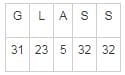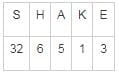Similarly,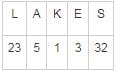RRB NTPC Mock Test - 2 (English) - Question 59

Who invented Electromagnetic Induction?

Detailed Solution for RRB NTPC Mock Test - 2 (English) - Question 59

Electromagnetic induction is the production of voltage or electromotive force due to a change in the magnetic field. Faraday discovered electromagnetic induction, the principle behind the electric transformer and generator.

RRB NTPC Mock Test - 2 (English) - Question 60

Mule is the hybrid of

Detailed Solution for RRB NTPC Mock Test - 2 (English) - Question 60

Mule is a hybrid between a male donkey and a female horse.

The scientific name of Mule is Equus mulus.

RRB NTPC Mock Test - 2 (English) - Question 61

If a mirror is placed on the line AB, then which of the answer figures is the right image of the given figure?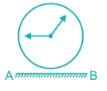Detailed Solution for RRB NTPC Mock Test - 2 (English) - Question 61

The mirror image of given figure is as follows,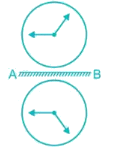Hence, correct answer is figure 3.

RRB NTPC Mock Test - 2 (English) - Question 62

If '+' means '×', '–' means '÷', '×' means '–' and '÷' means '+', then 26 – 52 + 74 = ?

Detailed Solution for RRB NTPC Mock Test - 2 (English) - Question 62

Given, '+' means '×', '–' means '÷', '×' means '–' and '÷' means '+'.

So,

26 − 52 + 74 → 26 ÷ 52 × 74

= 1/2 * 74

= 37

Thus, 37 is the correct alternative.

RRB NTPC Mock Test - 2 (English) - Question 63

In the following question, find out the alternative which will replace the question mark.
26th January : Indian Republic Day ∷ ? : World Water Day

Detailed Solution for RRB NTPC Mock Test - 2 (English) - Question 63

India on 26th January is observed as the Indian Republic Day.

Similarly, World water day is observed every year on every 22nd March.

Hence 22nd March is the answer.

RRB NTPC Mock Test - 2 (English) - Question 64

To go a certain distance of 40 km upstream a rower takes 8 hours while it takes her only 5 hours to row the same distance downstream. What was the rower’s speed in still water?

Detailed Solution for RRB NTPC Mock Test - 2 (English) - Question 64

Let x be the rower’s speed and y be the current speed of water.

Speed while going upstream = (x –y)

⇒ 40/ (x – y) = 8

⇒ x – y = 5      ---- (1)

Now speed while going downstream = (x + y)

⇒ 40/ (x + y) = 5

⇒ x + y = 8      ----(2)

From equation 1 and 2 we get

x = 6.5 km/hr

∴ Rower’s speed = 6.5 km/hr

RRB NTPC Mock Test - 2 (English) - Question 65

If 3 means 2, 10 means 16, 88 means 8, 74 means 4 and 99 means 45. What would be value of 88 + 99 - 74 × 10 ÷ 3?

Detailed Solution for RRB NTPC Mock Test - 2 (English) - Question 65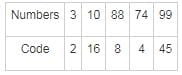Given: 88 + 99 - 74 × 10 ÷ 3

Using BODMAS

8 + 45 - 4 × 16 ÷ 2

8 + 45 - 4 × 8

8 + 45 - 32

= 21

Hence, the correct answer is 21.

RRB NTPC Mock Test - 2 (English) - Question 66

If a - 1/a = 1, then a2 + 1/a2 = ?

Detailed Solution for RRB NTPC Mock Test - 2 (English) - Question 66

Given that, a - 1/a = 1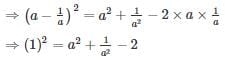∴ a2 + 1/a2 = 3

RRB NTPC Mock Test - 2 (English) - Question 67

Which among the following is used in making liquors, medicines and as a fuel in aircrafts?

Detailed Solution for RRB NTPC Mock Test - 2 (English) - Question 67

Ethyl alcohol is an important industrial chemical. It is used as a solvent, in the synthesis of other organic chemicals, and as an additive to automotive gasoline (forming a mixture known as a gasohol). It is present in a lot of things we use on a daily basis, ranging from perfumes to alcoholic beverages.

RRB NTPC Mock Test - 2 (English) - Question 68

80% of a number is 100. What is the number?

Detailed Solution for RRB NTPC Mock Test - 2 (English) - Question 68

Let the number be ‘x’.

80% of a number is 100,

⇒ x × 80/100 = 100

⇒ x = (100 × 100)/80 = 125

RRB NTPC Mock Test - 2 (English) - Question 69

Amoebic dysentery is caused by

Detailed Solution for RRB NTPC Mock Test - 2 (English) - Question 69
• Amoebic dysentery is an infection caused by Entamoeba histolytica
• The Symptoms may include abdominal pain, diarrhoea, or bloody diarrhoea.
• Prevention of Amoebic dysentery can be done by improving sanitation, including separating food and water from faeces.
RRB NTPC Mock Test - 2 (English) - Question 70

How much does one need to add to 2/3 to obtain 3/2?

Detailed Solution for RRB NTPC Mock Test - 2 (English) - Question 70

⇒ ? + 2/3 = 3/2

⇒ ? = 5/6

RRB NTPC Mock Test - 2 (English) - Question 71

Which of the following is used for making Rs. 1 coins?

Detailed Solution for RRB NTPC Mock Test - 2 (English) - Question 71

Rs. 1 Coin has the following characteristics-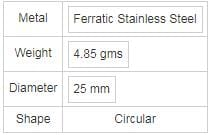Note: There are different sources which are giving different answers and The answer to this question can also be referred from the RBI website.

RRB NTPC Mock Test - 2 (English) - Question 72

The SI unit for impulse is:

Detailed Solution for RRB NTPC Mock Test - 2 (English) - Question 72

In classical mechanics, impulse is the integral of a force, F, over the time interval, t, for which it acts. Since force is a vector quantity, impulse is also a vector in the same direction. Its SI unit is Newton second.

RRB NTPC Mock Test - 2 (English) - Question 73

If the sum of two numbers is 25 and their product is 156. Find the larger number.

Detailed Solution for RRB NTPC Mock Test - 2 (English) - Question 73

Let the larger number be ‘x’

Smaller number = 25 - x

Product = x(25 - x) = 156

⇒ 25x - x2 = 156

⇒ x2 - 25x + 156 = 0

⇒ x2 - 12x - 13x + 156 = 0

⇒ x (x - 12) - 13(x - 12) = 0

⇒ (x - 12)(x - 13) = 0

⇒ x = 12, 13

Smaller number = 25 - x = 13, 12

∵ x is the larger number

∴ The larger number = 13

RRB NTPC Mock Test - 2 (English) - Question 74

Which Railway Station has become the first ever railway station in the Indian Railways to get an ISO certification from NGT for providing passenger amenities in a clean and green environment?

Detailed Solution for RRB NTPC Mock Test - 2 (English) - Question 74
• The Guwahati Railway Station has become the first ever railway station in the Indian Railways to get an ISO certification from the National Green Tribunal (NGT) for providing passenger amenities in a clean and green environment.
• The station also has amenities such as High-class reserve (VIP) lounge, waiting rooms, AC and non-AC retiring rooms, food courts and sanitation facilities.
RRB NTPC Mock Test - 2 (English) - Question 75

Vitamin C helps in the absorption of which of the following mineral?

Detailed Solution for RRB NTPC Mock Test - 2 (English) - Question 75

Vitamin C plays a pivotal role in the absorption of iron from various dietary sources.

RRB NTPC Mock Test - 2 (English) - Question 76

A thing or event in our environment which triggers our response is called:

Detailed Solution for RRB NTPC Mock Test - 2 (English) - Question 76

A stimulus is something that causes a physiological response.

RRB NTPC Mock Test - 2 (English) - Question 77

A series is given with one term missing. Select the correct alternative from the given ones that will complete the series.
31, 28, 31, 30, 31, 30, 31, ________, 30

Detailed Solution for RRB NTPC Mock Test - 2 (English) - Question 77

The relation among given numbers is the number of days in the Georgian calendar in the month:

1 → January → 31

2 → February → 28

3 → March → 31

4 → April → 30

5 → May → 31

6 → June → 30

7 → July → 31

8 → August → 31

9 → September → 30

Hence, “31” is the missing term.

RRB NTPC Mock Test - 2 (English) - Question 78

The common name of Magnesium Sulphate heptahydrate is

Detailed Solution for RRB NTPC Mock Test - 2 (English) - Question 78

Magnesium sulfate is an inorganic salt containing magnesium, sulfur and oxygen with the formula MgSO4. It is often encountered as the heptahydrate sulfate mineral epsomite commonly called Epsom salt.

RRB NTPC Mock Test - 2 (English) - Question 79

The focal length of a plane mirror is ______.

Detailed Solution for RRB NTPC Mock Test - 2 (English) - Question 79

The focal length of a plane mirror is infinity as the image can be formed at infinite distance inside the mirror.

RRB NTPC Mock Test - 2 (English) - Question 80

What is the unit digit of 1281 × 1387?

Detailed Solution for RRB NTPC Mock Test - 2 (English) - Question 80

Unit digit of 121 = 2

Unit digit of 122 = 4

Unit digit of 123 = 8

Unit digit of 124 = 6

Unit digit of 125 = 2

Same unit digit repeats after every 4th power

∴ Unit digit of 1281 = (124)20 × 12= 2

Unit digit of 131 = 3

Unit digit of 132 = 9

Unit digit of 133 = 7

Unit digit of 134 = 1

Unit digit of 135 = 3

Same unit digit repeats after every 4th power

∴ Unit digit of 1387 = (134)21 × 133 = 7

∴ Unit digit of 1281 × 1387 = unit digit of (2 × 7) = 4

RRB NTPC Mock Test - 2 (English) - Question 81

Find the mean proportion between 16 and 25.

Detailed Solution for RRB NTPC Mock Test - 2 (English) - Question 81

Let the mean proportion be x

Therefore, 16 : x = x : 25

⇒ x × x = 16 × 25

⇒ x2 = 400

⇒ x = 20

RRB NTPC Mock Test - 2 (English) - Question 82

Cristiano Ronaldo is related to which country?

Detailed Solution for RRB NTPC Mock Test - 2 (English) - Question 82

Ronaldo is a Portuguese professional footballer who plays for Spanish club Real Madrid and the Portugal national team. He is a forward and serves as captain for Portugal.

RRB NTPC Mock Test - 2 (English) - Question 83

What happens when an electric current is passed through a wire?

Detailed Solution for RRB NTPC Mock Test - 2 (English) - Question 83

When a current passes through a wire, a part of the electrical energy is converted into heat energy as a result of some resistance experienced by it and as a result the wire gets heated. This is known as heating effect of electric current.

RRB NTPC Mock Test - 2 (English) - Question 84

Detailed Solution for RRB NTPC Mock Test - 2 (English) - Question 84
• Environmental degradation is the deterioration of the environment through depletion of resources, the destruction of ecosystems, habitat destruction, the extinction of wildlife, and pollution.
• Thus it means overall lowering of environmental qualities
• It also means adverse change brought by human activities
• And it causes ecological imbalance.
RRB NTPC Mock Test - 2 (English) - Question 85

Direction: Study the table given below and answer the following question.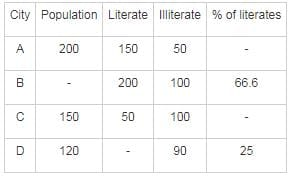Based on the given data, the percentage of literates in city A is _________.

Detailed Solution for RRB NTPC Mock Test - 2 (English) - Question 85

Population of city A = 200

No. of literates in city A = 150

∴ Percentage of literates in city A = 150/200 × 100 = 75%

RRB NTPC Mock Test - 2 (English) - Question 86

Find value of (62% of 650) + (45% of 340).

Detailed Solution for RRB NTPC Mock Test - 2 (English) - Question 86

(62% of 650) + (45% of 340) = (62/100) × 650 + (45/100) × 340 = 556

RRB NTPC Mock Test - 2 (English) - Question 87

Find the standard deviation of 15, 20, 18, 22, 25.

Detailed Solution for RRB NTPC Mock Test - 2 (English) - Question 87

Given Number are 15, 20, 18, 22 and 25

⇒ Mean = (15 + 20 + 18 + 22 + 25)/5 = 100/5 = 20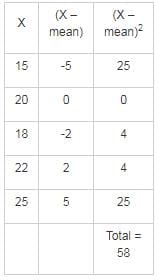⇒ Standard deviation = √(58/5) = √11.6

RRB NTPC Mock Test - 2 (English) - Question 88

Who among the following actresses played the role of Priyanka Gandhi in movie  Accidental Prime Minister?

Detailed Solution for RRB NTPC Mock Test - 2 (English) - Question 88

Actress Aahana Kumra, who is known for her work in the TV industry, is playing Priyanka Gandhi in The Accidental Prime Minister.

RRB NTPC Mock Test - 2 (English) - Question 89

Solve the following.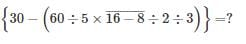Detailed Solution for RRB NTPC Mock Test - 2 (English) - Question 89By BODMAS,

⇒ {30 – (12 × 8 ÷ 2 ÷ 3)} = ?

⇒ {30 – (12 × 4 ÷ 3)} = ?

⇒ {30 – (12 × 4 ÷ 3)} = ?

∴ ? = 14

RRB NTPC Mock Test - 2 (English) - Question 90

An article having marked price of Rs. 4300 is sold at a discount of 17%. What is the selling price (in Rs.) of the article?

Detailed Solution for RRB NTPC Mock Test - 2 (English) - Question 90

Marked Price = 4300

Discount = 17%

⇒ SP = 4300 - 0.17 × 4300 = 3569

∴ Selling Price = Rs. 3569

RRB NTPC Mock Test - 2 (English) - Question 91

A train, 200 meters long, is running at a speed of 30 meter per second. It will cross a bridge of 523 meters long in ... seconds

Detailed Solution for RRB NTPC Mock Test - 2 (English) - Question 91

Train length = 200 meter

Train speed = 30 m/s

Length of bridge = 523 meter

According to the question,

∴ Time taken by a 200 m long train to cross a bridge of 523 m

⇒ (523 + 200)/30 = 24.1 seconds

RRB NTPC Mock Test - 2 (English) - Question 92

A piece of paper is folded and punched as shown below in the question figures. From the given answer figures, indicate how it will appear when opened.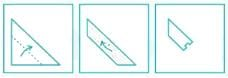Detailed Solution for RRB NTPC Mock Test - 2 (English) - Question 92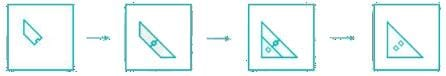Hence, option 1 is the correct figure.

RRB NTPC Mock Test - 2 (English) - Question 93

The amount received at 10% per annum compound interest after 3 yrs is Rs. 10,648. What was the principal (in Rs.)?

Detailed Solution for RRB NTPC Mock Test - 2 (English) - Question 93

We have,

Amount = Principal × (1 + Rate/100)time

⇒ 10648 = Principal × (1 + 10/100)3

⇒ 10648 = 1.331 × Principal

⇒ Principal = 10648/1.331 = 8000

RRB NTPC Mock Test - 2 (English) - Question 94

In which year was the first electric train rolled in India?

Detailed Solution for RRB NTPC Mock Test - 2 (English) - Question 94

The first electric train ran in India started with the inauguration of services between Bombay VT and Kurla Harbour on 3rd Feb 1925 on ex-GIP Railway system.

RRB NTPC Mock Test - 2 (English) - Question 95

The green colours seen in the fireworks is due to the presence of ________.

Detailed Solution for RRB NTPC Mock Test - 2 (English) - Question 95
• Barium is used to create green colours in fireworks.
• The Strontium salts impart a Crimson Red colour to the fireworks.
• Strontium imparts a Crimson Red colour because when the excited atoms of Strontium contained in the salt return to the ground state it imparts electromagnetic radiation which is seen by the viewer as an emitted colour.
• Sodium imparts a yellow colour to the fireworks while barium imparts a green colour.
RRB NTPC Mock Test - 2 (English) - Question 96

A, B and C can complete a task together in 8 days. A and B would need 16 days if only the two of them worked, while A and C would take 12 days if only the two of them worked. If A worked alone, how many days would A take to compete the task?

Detailed Solution for RRB NTPC Mock Test - 2 (English) - Question 96

(A + B + C) can complete a work in = 8 days

⇒ (A + B + C)’s 1 day’s work = 1/8

(A + B) can complete a work in = 16 days

⇒ (A + B)’s 1 day’s work = 1/16

⇒ C’s 1 day’s work = 1/8 – 1/16 = 1/16

(A + C) can complete a work in = 12 days

⇒ (A + C)’s 1 day’s work = 1/12

⇒ A’s 1 day’s work = 1/12 – 1/16 = 1/48

∴ A takes 48 days to complete the work alone.

RRB NTPC Mock Test - 2 (English) - Question 97

How many brackets would you remove from the equation to make it correct?
{(16 - 8 + 2 × 4) ÷ 8 - 9 - 3 + 6} × 5 = (32 + 4) × 3 + 12 - 105

Detailed Solution for RRB NTPC Mock Test - 2 (English) - Question 97

Removing inside bracket in LHS will satisfy the equation.

Given equation: {(16 - 8 + 2 × 4) ÷ 8 - 9 - 3 + 6} × 5 = (32 + 4) × 3 + 12 - 105

After removing inside bracket of LHS we get,

(16 - 8 + 2 × 4 ÷ 8 - 9 - 3 + 6) × 5 = (32 + 4) × 3 + 12 - 105

(16 - 8 + 2 × 1 ÷ 2 - 9 - 3 + 6) × 5 = 36 × 3 + 12 - 105

(16 - 8 + 1 - 9 - 3 + 6) × 5 = 36 × 3 + 12 - 105

(16 + 1 + 6 - 8 - 9 - 3) × 5 = 36 × 3 + 12 - 105

(23 - 20) × 5 = 36 × 3 + 12 - 105

(23 - 20) × 5 = 108 + 12 - 105

3 × 5 = 120 - 105

15 = 15

LHS = RHS

Hence option 1) is correct.

RRB NTPC Mock Test - 2 (English) - Question 98

Rotavac vaccine protects against which of the following diseases?

Detailed Solution for RRB NTPC Mock Test - 2 (English) - Question 98

The Rotavac vaccine, developed by the Bharat Biotech Limited, was included in India’s national immunisation programme. Rotavac vaccine protects against childhood diarrhoea caused by rotavirus infections.

RRB NTPC Mock Test - 2 (English) - Question 99

Directions: A statement is given followed by two inferences I and II. You have to consider the statement to be true even if it seems to be at variance from commonly known facts. You have to decide which of the given inferences, if any, follow from the given statement.

Statement:
Exercising daily is recommended for good physical and mental health.

Inferences:
1. Exercising alone will keep one disease free.
2. Exercising daily, refreshes body and mind and helps us stay fit.

Detailed Solution for RRB NTPC Mock Test - 2 (English) - Question 99

It is can be understood that exercising is good for mind and body but exercising solely cannot keep one disease free. Thus, inference I does not follow.

We know that the statement speaks of physical and mental health, thus it can be inferred that exercising daily will refresh the mind and body keeping it healthy and fit.

Thus, only inference II follows and option 2 is correct.

RRB NTPC Mock Test - 2 (English) - Question 100

Average of 31 numbers is 19. If the average of first 8 numbers is 24, then what is the average of the remaining numbers?

Detailed Solution for RRB NTPC Mock Test - 2 (English) - Question 100

Average of 31 number = Sum of 31 number/31

⇒ 19 = sum of 31 number/31

⇒ Sum of 31 number = 589

Now,

Average of 8 numbers = sum of 8 number/8

⇒ 24 = sum of 8 numbers/8

⇒ Sum of 8 numbers = 192

Now,

Sum of remaining numbers = 589 - 192 = 397

∴ Average = 397/23 = 17.26

## RRB NTPC Mock Tests Series

20 tests
 Use Code STAYHOME200 and get INR 200 additional OFF Use Coupon Code
Information about RRB NTPC Mock Test - 2 (English) Page
In this test you can find the Exam questions for RRB NTPC Mock Test - 2 (English) solved & explained in the simplest way possible. Besides giving Questions and answers for RRB NTPC Mock Test - 2 (English), EduRev gives you an ample number of Online tests for practice

20 tests